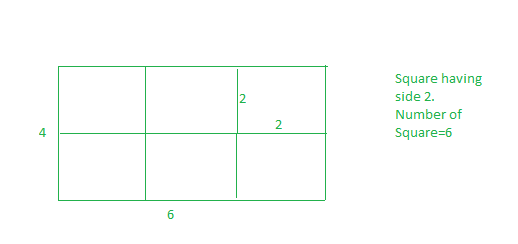Skip to content
Related Articles
Minimum squares to evenly cut a rectangle
• Difficulty Level : Easy
• Last Updated : 23 Apr, 2021

Given a rectangular sheet of length l and width w. we need to divide this sheet into square sheets such that the number of square sheets should be as minimum as possible.
Examples:

Input :l= 4 w=6
Output :6
We can form squares with side of 1 unit, But the number of squares will be 24, this is not minimum. If we make square with side of 2, then we have 6 squares. and this is our required answer.
And also we can’t make square with side 3, if we select 3 as square side, then whole sheet can’t be converted into suares of equal length.Input :l=3 w=5
Output :15

Optimal length of the side of a square is equal to GCD of two numbers

## C++

 `// CPP program to find minimum number of``// squares to make a given rectangle.``#include ``using` `namespace` `std;` `int` `countRectangles(``int` `l, ``int` `w)``{``    ``// if we take gcd(l, w), this``    ``// will be largest possible``    ``// side for suare, hence minimum``    ``// number of square.``    ``int` `squareSide = __gcd(l, w);` `    ``// Number of squares.``    ``return` `(l * w) / (squareSide * squareSide);``}` `// Driver code``int` `main()``{``    ``int` `l = 4, w = 6;``    ``cout << countRectangles(l, w) << endl;``    ``return` `0;``}`

## Java

 `// Java program to find minimum number of``// squares to make a given rectangle.` `class` `GFG{``static` `int` `__gcd(``int` `a, ``int` `b) {``   ``if` `(b==``0``) ``return` `a;``   ``return` `__gcd(b,a%b);``}``static` `int` `countRectangles(``int` `l, ``int` `w)``{``    ``// if we take gcd(l, w), this``    ``// will be largest possible``    ``// side for suare, hence minimum``    ``// number of square.``    ``int` `squareSide = __gcd(l, w);` `    ``// Number of squares.``    ``return` `(l * w) / (squareSide * squareSide);``}` `// Driver code``public` `static` `void` `main(String[] args)``{``    ``int` `l = ``4``, w = ``6``;``    ``System.out.println(countRectangles(l, w));``}``}``// This code is contributed by mits`

## Python3

 `# Python3 code to find minimum number of``# squares to make a given rectangle.` `import` `math` `def` `countRectangles(l, w):` `    ``# if we take gcd(l, w), this``    ``# will be largest possible``    ``# side for suare, hence minimum``    ``# number of square.``    ``squareSide ``=` `math.gcd(l,w)``    ` `    ``# Number of squares.``    ``return` `(l``*``w)``/``(squareSide``*``squareSide)` `# Driver Code``        ` `if` `__name__ ``=``=` `'__main__'``:``    ``l ``=` `4``    ``w ``=` `6``    ``ans ``=` `countRectangles(l, w)``    ``print` `(``int``(ans))` `# this code is contributed by``# SURENDRA_GANGWAR`

## C#

 `// C# program to find minimum number of``// squares to make a given rectangle.` `class` `GFG{``static` `int` `__gcd(``int` `a, ``int` `b) {``if` `(b==0) ``return` `a;``return` `__gcd(b,a%b);``}``static` `int` `countRectangles(``int` `l, ``int` `w)``{``    ``// if we take gcd(l, w), this``    ``// will be largest possible``    ``// side for suare, hence minimum``    ``// number of square.``    ``int` `squareSide = __gcd(l, w);` `    ``// Number of squares.``    ``return` `(l * w) / (squareSide * squareSide);``}` `// Driver code``public` `static` `void` `Main()``{``    ``int` `l = 4, w = 6;``    ``System.Console.WriteLine(countRectangles(l, w));``}``}``// This code is contributed by mits`

## PHP

 ``

## Javascript

 ``
Output:
`6`

Attention reader! Don’t stop learning now. Get hold of all the important DSA concepts with the DSA Self Paced Course at a student-friendly price and become industry ready.  To complete your preparation from learning a language to DS Algo and many more,  please refer Complete Interview Preparation Course.

In case you wish to attend live classes with industry experts, please refer Geeks Classes Live

My Personal Notes arrow_drop_up